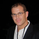26415 views
Variable Moving Average, often abbreviated as VMA, is an Exponential Moving Average developed by Tushar S. Chande. VMA automatically adjusts its smoothing constant on the basis of Market Volatility .

Use this like other Moving Averages. I have added the following options that can be enabled via options page:
- Trend Direction Indication: Green = Up trend, Blue = Potential congestion, Red = down trend.
- Color bars based on Trend

List of my other indicators:
- Chart:
//
// @author LazyBear
// List of all my indicators:
//
study(title="Variable Moving Average [LazyBear]", shorttitle="VMA_LB", overlay=true)
src=close
l =input(6, title="VMA Length")
std=input(false, title="Show Trend Direction")
bc=input(false, title="Color bars based on Trend")
k = 1.0/l
pdm = max((src - src), 0)
mdm = max((src - src), 0)
pdmS = ((1 - k)*nz(pdmS) + k*pdm)
mdmS = ((1 - k)*nz(mdmS) + k*mdm)
s = pdmS + mdmS
pdi = pdmS/s
mdi = mdmS/s
pdiS = ((1 - k)*nz(pdiS) + k*pdi)
mdiS = ((1 - k)*nz(mdiS) + k*mdi)
d = abs(pdiS - mdiS)
s1 = pdiS + mdiS
iS = ((1 - k)*nz(iS) + k*d/s1)
hhv = highest(iS, l)
llv = lowest(iS, l)
d1 = hhv - llv
vI = (iS - llv)/d1
vma = (1 - k*vI)*nz(vma) + k*vI*src
vmaC=(vma > vma) ? green : (vma<vma) ? red : (vma==vma) ? blue : black
plot(vma, color=std?vmaC:black, linewidth=3, title="VMA")
barcolor(bc?vmaC:na)
List of my free indicators: http://bit.ly/1LQaPK8
List of my indicators at Appstore: http://blog.tradingview.com/?p=970

## Kommentare